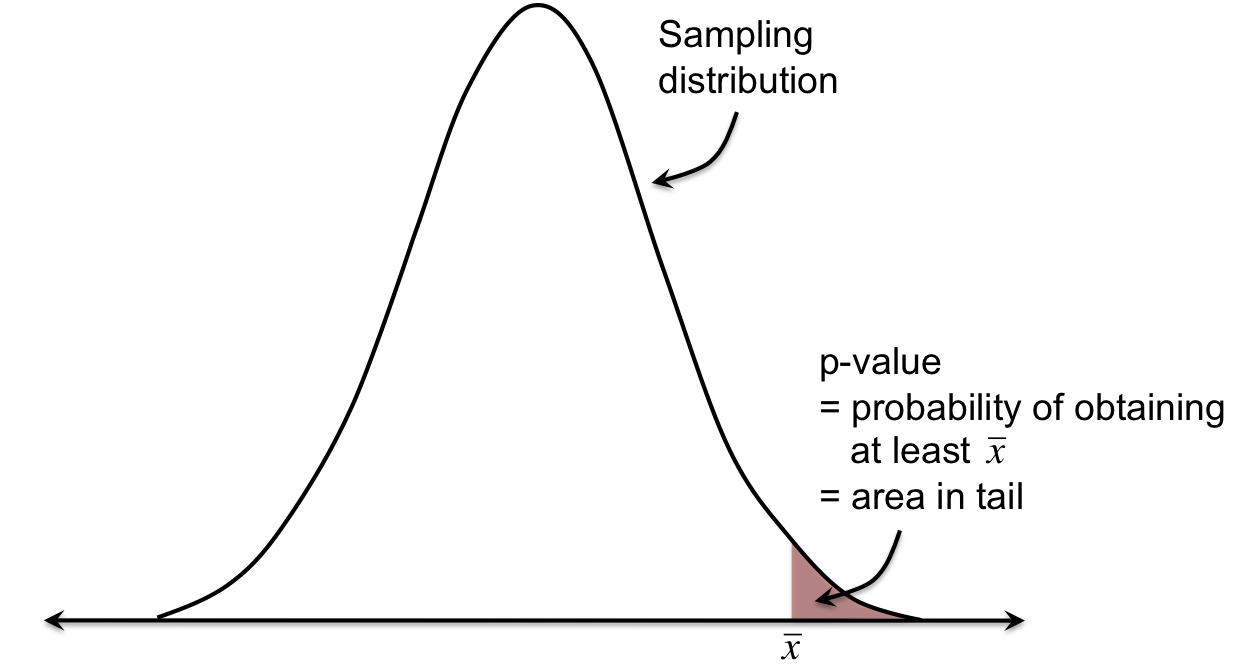You’ve now seen two ways of judging whether or not a sample mean is likely or unlikely. The first is by looking at where the sample mean falls on the sampling distribution in relation to the population mean. The second is by looking at the confidence interval (typically a 95% CI) for the true population mean if everyone were to have that intervention (μI), and see if the original population mean falls in this range.

In this lesson, we’ll focus again on the first method—where a sample mean falls on the sampling distribution—and formalize the procedure of deciding whether or not this sample mean is likely or unlikely. We do this by finding the p-value, which is the probability of obtaining that sample mean.If x̅ is greater than μ, the p-value is the probability of obtaining a sample mean of at least x̅.If x̅ is less than μ, the p-value is the probability of obtaining a sample mean of at most x̅.

Oftentimes, we decide that a sample mean is significantly unlikely if the p-value is less than 0.05 (called our alpha level, or significance level). Let’s do an example.

This is a preview of Lesson 9. To access the full book, please purchase a hard copy or a digital version. If you opt for the digital version, you will receive a link via email within 1 business day.

Continue to Lesson 10, or select a lesson below.